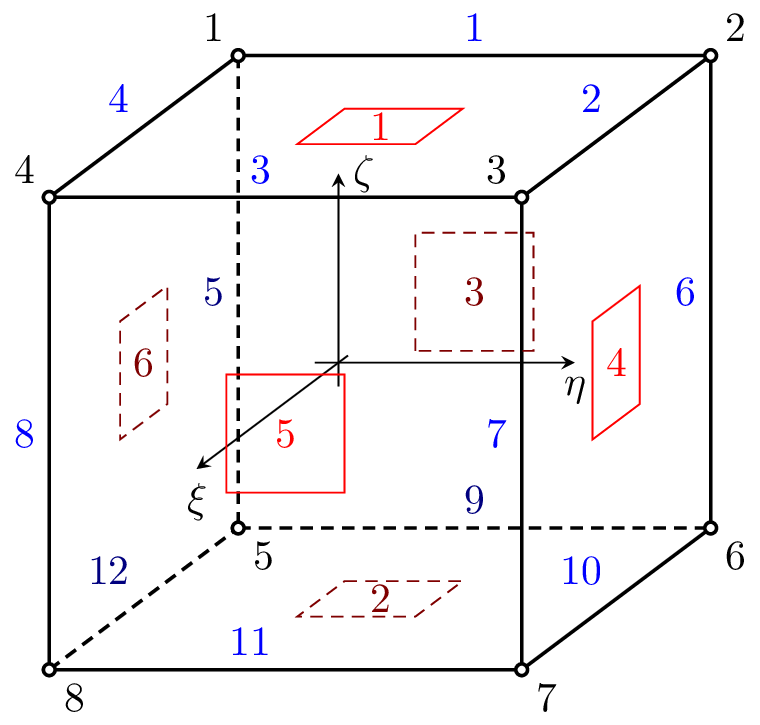#### 4.2 2D CBS Elements

##### 4.2.1 Tr1CBS element

Represents the linear triangular finite element for transient incompressible flow analysis using cbs algorithm with equal order approximation of velocity and pressure fields. Each node has 3 degrees of freedoms (two components of velocity and pressure). The node numbering is anti-clockwise. The element features are summarized in Table 60.Figure 25: Tr1CBS element. Node numbering, Side numbering and definition of local edge c.s.(a).

 Keyword Tr1CBS Description linear triangular finite element for transient incompressible flow analysis using cbs algorithm Specific parameters [bsides #(ia)] [bcodes #(ia)] Parameters Since the problem formulation requires to evaluate some boundary terms, the element boundary edges should be specified as well as the types of boundary conditions applied at these boundary edges. The boundary edges (their numbers) are specified using bsides array. The type of boundary condition(s) applied to corresponding boundary side is determined by bcodes array. The available/supported boundary codes are following: 1 for prescribed traction, 2 for prescribed normal velocity, 4 for prescribed tangential velocity, and 8 for prescribed pressure. If the element side is subjected to a combination of these fundamental types boundary conditions, the corresponding code is obtained by summing up the corresponding codes. Unknowns Two velocity components (V_u and V_v) and pressure (P_f) are required in each node. Approximation Equal order approximation of velocity and pressure fields. Integration exact Features Constant boundary tractions are supported1 . Body loads representing the self-weight load are supported. Status Untested

Table 60: Tr1CBS element summary